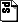## Graph Nonisomorphism Has Subexponential Size Proofs Unless The Polynomial-Time Hierarchy Collapses#### Abstract

Traditional hardness versus randomness results focus on time-efficient randomized decision procedures. We generalize these trade-offs to a much wider class of randomized processes. We work out various applications, most notably to derandomizing Arthur-Merlin games. We show that every language with a bounded round Arthur-Merlin game has subexponential size membership proofs for infinitely many input lengths unless exponential time coincides with the third level of the polynomial-time hierarchy (and hence the polynomial-time hierarchy collapses). Since the graph nonisomorphism problem has a bounded round Arthur-Merlin game, this provides the first strong evidence that graph nonisomorphism has subexponential size proofs.

For a randomized procedure to fit within our framework, we only require that for any fixed input the complexity of checking whether the procedure succeeds on a given random bit sequence is not too high. We apply our derandomization technique to four other complexity theoretic constructions:

• The Valiant-Vazirani random hashing technique which prunes the number of satisfying assignments of a Boolean formula to one, and related procedures like computing satisfying assignments to Boolean formulas non-adaptively given access to an oracle for satisfiability.
• The algorithm of Bshouty et al. for learning Boolean circuits.
• Constructing matrices with high rigidity.
• Constructing polynomial-size universal traversal sequences.

We also establish hardness versus randomness tradeoffs for space bounded computation.

dieter@cs.wisc.edu